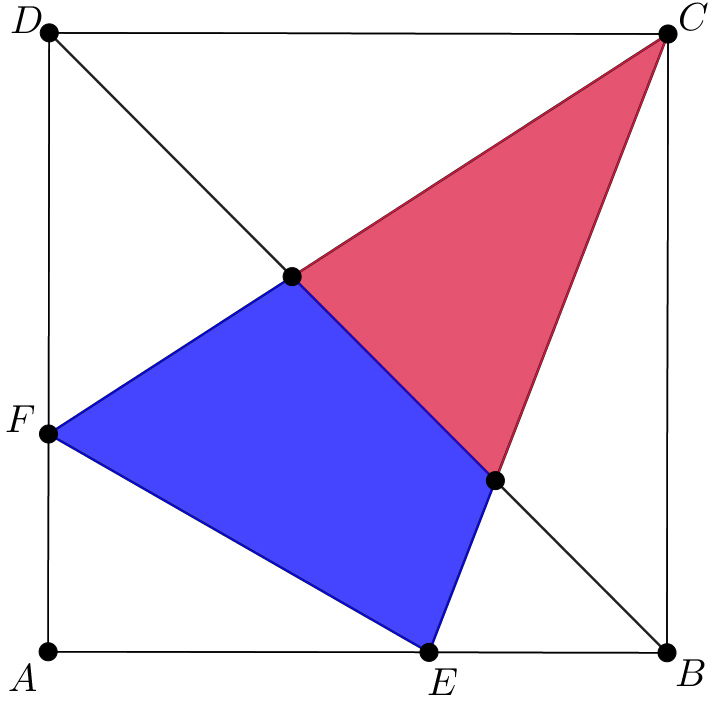# Try it! Just a little geometry

Geometry Level 3$ABCD$ is a square which has an area of $1$. $E$ and $F$ are points on the square's sides as shown in the figure above. $\Delta AEF$ has a perimeter of $2$. Diagonal $BD$ divides $\Delta ECF$ into two pieces: a smaller triangle and a quadrilateral. Which has a larger area?

×